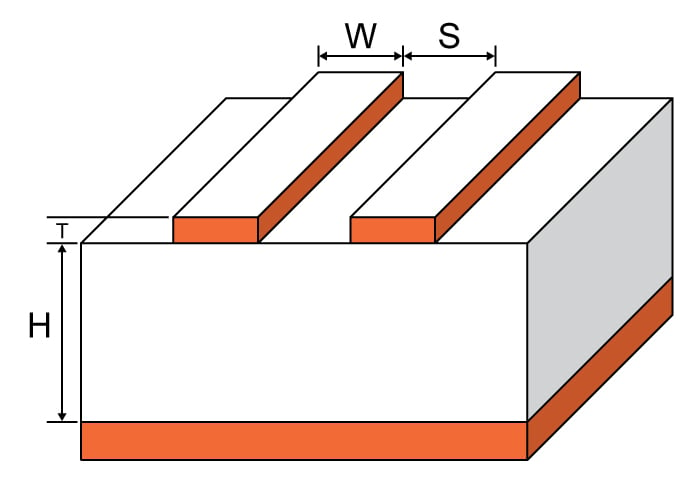# Edge Coupled Microstrip Impedance Calculator

## This tool helps calculate the impedance of an edge-coupled microstrip.

Ohms
Ohms
Ohms
Ohms

### Overview

This calculator is designed to calculate the characteristic impedance of an edge-coupled microstrip. Such a microstrip is constructed with two traces referenced to the same reference plane with a dielectric material between them. One of the features of this type of microstrip is the coupling between lines.

To use this tool, enter the values for trace thickness, substrate height, trace width, trace spacing, and dielectric constant in the calculator above and press the "calculate" button. The tool generates odd, even, common, and differential impedance as output values. See below for the definition of these impedances. The default unit for all given values, except the dielectric constant, is mils. It is possible to select other units.

The calculator is appropriate for use when the following are all true:

$$0.1 \le u = \frac{w}{h} \le 10$$

$$0.1 \le g = \frac{s}{h} \le 10$$

$$1 \le e_r \le 18$$### Constants

Free space characteristic impedance: $$\eta_0 = 376.73 \space \Omega$$

Euler's number: $$e = 2.71828$$

### Equations

In the below equations, "er" refers to εr (i.e., the dielectric constant, also known as relative permittivity).

$$Z_{0_{odd}}=Z_{0_{surf}}\cdot \left [ \frac{\sqrt{\frac{er_{eff}}{er_{effo}}}}{1-\left ( \frac{zo_{surf}}{\eta_{o}}\cdot q_{10}\sqrt{er_{eff}} \right )} \right ]$$

$$Z_{0_{even}}=Z_{0_{surf}}\cdot \frac{\sqrt{\frac{er_{eff}}{er_{eff,e}}}}{1-\frac{zo_{surf}}{\eta_{o}}\cdot q_{4}\cdot \sqrt{er_{eff}}}$$

When $$\frac{w}{h} < 1$$, the effective dielectric constant is calculated as:

$$er_{eff1}=\frac{er+1}{2}+\left ( \frac{er-1}{2} \right )\cdot \left ( \sqrt{\frac{w}{w+12h}}+0.04\left ( 1-\frac{w}{h} \right )^{2} \right )$$

When $$\frac{w}{h} \ge 1$$, the effective dielectric constant is calculated as:

$$er_{eff2}=\frac{er+1}{2}+\left ( \frac{er-1}{2} \right )\cdot \left ( \sqrt{\frac{w}{w+12h}} \right )$$

The remaining equations are as follows:

$$Z_{0_{surf}}=\frac{\eta_{o}}{2\pi \sqrt{2}\sqrt{er_{eff}+1}}\cdot \ln \left ( 1+\left ( 4\cdot \frac{h}{w_{eff}} \right )\cdot \left (\left ( 4\cdot \frac{h}{w_{eff}} \right )\cdot\left ( \frac{14\cdot er_{eff}+8}{11\cdot er_{eff}} \right )+ temp \right )\right )$$

$$a_{0}=0.7287\left ( er_{eff}-\frac{er+1}{2} \right )\cdot \left ( \sqrt{1-e^{-0.179u}} \right )$$

$$b_{0}=\frac{0.747\cdot er}{0.15+er}$$

$$c_{0}=b_{0}-\left ( b_{0}-0.207 \right )\cdot e^{-0.414u}$$

$$d_{0}=0.593+0.694e^{-0.562u}$$

$$g=\frac{s}{h}$$

$$w_{eff}=w+\frac{t}{\pi }\cdot \ln \left ( \frac{4e}{\sqrt{\left ( \frac{t}{h} \right )^{2}+\left ( \frac{t}{w\pi +1.1t\pi } \right )^{2}}} \right )\cdot \frac{er_{eff}+1}{2\cdot er_{eff}}$$

$$er_{effo}=\left ( \left ( 0.5\cdot \left ( er+1 \right )+a_{0}-er_{eff} \right )\cdot e^{-c_{0}\cdot g^{d_{0}}} \right )+er_{eff}$$

$$temp=\sqrt{16\left ( \frac{h}{w_{eff}} \right )^{2}\cdot \left ( \frac{14\cdot er_{eff}+8}{11\cdot er_{eff}} \right )^{2}+\left ( \frac{er_{eff}+1}{2er_{eff}} \right )\cdot \pi ^{2}}$$

$$q_{1}=0.8695\cdot u^{0.194}$$

$$q_{2}=1+0.7519\cdot g+1.89g^{2.31}$$

$$q_{3}=0.1975+\left ( 16.6+\left ( \frac{8.4}{g} \right )^{6} \right )^{-0.387}+\frac{1}{241} \cdot \ln \left ( \frac{g^{10}}{1+\left ( \frac{g}{3.4} \right )^{10}} \right )$$

$$q_{4}=\frac{2\cdot q_{1}}{q_{2}\left ( e^{-g}\cdot u^{q_{3}}+\left ( 2-e^{-g} \right )\cdot u^{-q_{3}} \right )}$$

$$q_{5}=1.794+1.14\cdot \ln \left ( 1+\left ( \frac{0.638}{g+0.517\cdot g^{2.43}} \right ) \right )$$

$$q_{6}=0.2305+\frac{1}{281.3}\cdot \ln \left ( \frac{g^{10}}{1+\left ( \frac{g}{5.8} \right )^{10}} \right )+\frac{1}{5.1}\cdot \ln \left ( 1+0.598\cdot g^{1.154} \right )$$

$$q_{7}=\frac{10+190\cdot g^{2}}{1+82.3\cdot g^{3}}$$

$$q_{8}=e^{\left(-6.5 -0.95\cdot\ln (g) -\left (\frac{g}{0.15}\right )^{5}\right)}$$

$$q_{9}=\ln \left ( q_{7} \right )\cdot \left ( q_{8}+\frac{1}{16.5} \right )$$

$$q_{10}=\left ( \frac{1}{q_{2}} \right )\cdot \left ( q_{2}\cdot q_{4}-q_{5}\cdot e^{\left ( \ln\left ( u \right )\cdot q_{6}\cdot u^{-q_{9}} \right )} \right )$$

$$v=\frac{u\cdot \left ( 20+g^{2} \right )}{10+g^{2}}+ge^{-g}$$

$$ae(v)=1+\frac{\ln \left ( \frac{v^{4}+\left ( \frac{v}{52} \right )^{2}}{v^{4}+0.432} \right )}{49}+\frac{\ln \left ( 1+\left ( \frac{v}{18.1} \right )^{3} \right )}{18.7}$$

$$b_{e}(e_{r})=0.564\left ( \frac{er-0.9}{er+3} \right )^{0.053}$$

$$er_{eff,e}=\frac{er+1}{2}+\frac{er-1}{2}\cdot \left ( 1+\frac{10}{v} \right )^{-ae(v)\cdot b_{e}(e_{r})}$$

### Notes:

Odd Impedance ($$Z_{0_{odd}}$$): The impedance between one differential trace and the ground plane with the differential signals are driven with opposite polarity.

$$Z_{0_{odd}}=\frac{Z_{0_{diff}}}{2}$$

Even Impedance ($$Z_{0_{even}}$$): The impedance between one differential trace and the ground plane with the differential signals are driven with the same signal.

$$Z_{0_{even}} = 2Z_{0_{common}}$$

Differential Impedance ($$Z_{0_{diff}}$$): The impedance between the two lines with opposite polarity signals.

$$Z_{0_{diff}} = 2Z_{0_{odd}}$$

Common Impedance ($$Z_{0_{common}}$$): The impedance between the two lines with the same signal.

$$Z_{0_{common}}=\frac{Z_{0_{even}}}{2}$$

### Applications

Microwave antennas and couplers as well as some filters can be created using the edge-coupled microstrip. These transmission lines are popular because they can be manufactured cheaper than the traditional waveguide and are also more portable. The disadvantage of edge-coupled microstrips is their limited power-handling capabilities. Other issues with such transmission lines are high power loss, cross-talk, and unintentional radiation. Edge-coupled microstrips also find themselves in high-speed digital PCB design where differential signals are handled.• T
tome.matos September 19, 2019

Hi,

How do you calculate ereff? Is it based on ereff1 and ereff2?

Thank you.

Tomé

Like.
•SHF February 18, 2021

Hi, there are some weird points in the equations used here:
1. Z0_surf here is the single microstrip impedance proposed by Wheeler. I though the dielectric constant should be “er” rather than “er_eff”
Also, the thickness correction should be avoided because the origin paper only discussed the zero-thickness case.
-> Origin paper: https://ieeexplore.ieee.org/document/1132616?denied=
If the finite-thickness is desired, you can see Jansen’s work, which proposed suitable finite-thickness correction to the coupled microstrip line.
-> Finite thickness correction: https://ieeexplore.ieee.org/document/1129316?denied=
2. All the “er_eff” in the formulae, not “er_eff_o” and “er_eff_e”, should be “er”
3. The effective dielectric constant in the even mode is wrong because there was a typo in the first publish. After that, the authors have fixed it in the correction
paper.
->  correction paper: https://ieeexplore.ieee.org/document/1133005?denied=
Owing to not knowing your algorithm, I just took the formulae you used as the rules you programmed.

Like.
•SHF February 18, 2021
Hopes for your revision to the calculators because the calculated impedance by commercial soft wares and calculator here are rather different. The errors could exceed 10%, so I'd like to give some advice to you.
Like.